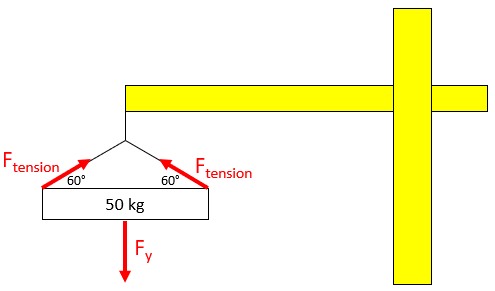## Tensional Force

Consider a construction crane is suspending a 50 kg payload in the shown diagram. What is the tensional force in each of the two cables holding up the load?Hint
The problem is asking to solve for the y-component of $$F_{tension}$$ in the free body diagram:Hint 2
To find $$F_y$$ , multiply the mass by gravity (Newton's 2nd law):
$$F_y=mass\times acceleration$$$The problem is asking to solve for the y-component of $$F_{tension}$$ in the free body diagram:To find $$F_y$$ , multiply the mass by gravity (Newton's 2nd law): $$F_y=mass\times acceleration=50kg\times 9.8m/s^2=490\:N$$$
Because there are two upward pulling cables in the vertical direction, they will counteract the entire downward force. Thus:
$$F_y=(2)F_{tension}\cdot sin(60^{\circ})=490\:N$$$$$F_{tension}=\frac{490N}{2\cdot sin(60^{\circ})}=\frac{490N}{1.732}=283\:N$$$
283 N